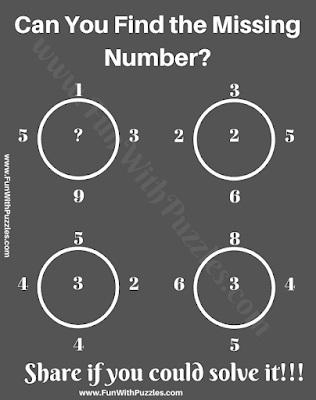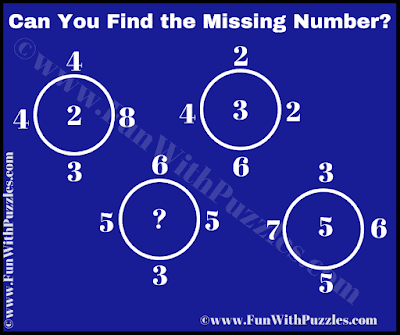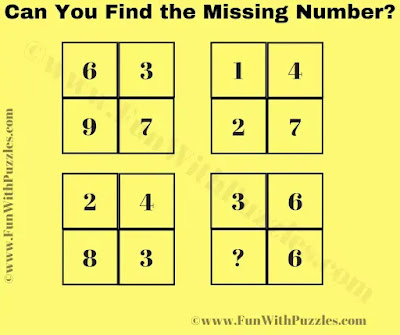These are the logical maths number puzzles for students which will challenge your brain. In these brain-challenging number puzzles, you are shown some numbers. Numbers that are given in each puzzle image, related to each other. Your challenge in these puzzles is to crack the logical pattern which is used in each puzzle to relate the given numbers. Once you are able to crack the logic, find the value of the missing number. Can you find the Number which replaces the question mark in each puzzle question?1. Which Number Replaces the Question Mark?2. Which Number Replaces the Question Mark?3. Which Number Replaces the Question Mark?4. Which Number Replaces the Question Mark?5. Which Number Replaces the Question Mark?

The answer to this "....", can be viewed by clicking on the answer button.

Unknown said...

Q1)
(a*a)+(d*d)=bc
3*3+4*4=25
.
.
.
And so on

Anonymous said...